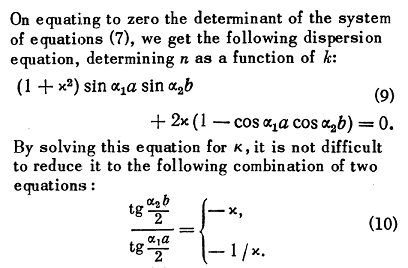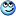# Trying to understand a derivation in a paper

Hello PF,
first of all I don't know where to put this post as it's not exactly a homework question but a clarification question for a project.
I'm going through the derivation of the effective permeability of two stacked medias, given the polarization of an incoming EM wave but I'm stuck at the point shown in the picture.
The title of the paper is "Electromagnetic Properties of a finely Stratified Medium" by S.M. Rytov in 1956 (I can share it if it's needed).

## Homework Statement## Homework Equations

$$\frac{sin(x)}{cos(x)} = tg(x)$$

## The Attempt at a Solution

I don't understand the notation they use and how they solve equation (9).
Does it mean $$\frac{tg\frac{b\alpha_2}{2}}{tg\frac{a\alpha_1}{2}}$$ is equal to $-\chi$ and $-1/\chi$? Or how is it supposed to be understood?Regarding how to solve the equation the closest I get is this: $$\frac{1+\chi^2}{2\chi}\ \ tg(a\alpha_1)\ \ tg(b\alpha_2)+(cos(a\alpha_1)\ \ cos(b\alpha_2))^{-1} = 1$$

#### Attachments

BvU
Homework Helper
the closest I get is this
(Is that how you solve a quadratic equation ?
(9) is of the form ##a\kappa^2 + b\kappa + c = 0 ## and you are supposed to be able to solve for ##\kappa##Last edited:
•Homework Helper
Gold Member
I think I got it to work.
Suggestion: Let ##a \alpha_1=\theta ## and ## b \alpha_2=\phi ##. You then get:
## x=\frac{-2(1-cos(\theta)cos(\phi)) \pm 2\sqrt{(cos(\theta)-cos(\phi))^2}}{2 sin(\theta)sin(\phi)} ##.
(This was obtained with the quadratic formula, (## x=\frac{-b \pm \sqrt{b^2-4ac}}{2a} ##, when starting with ## ax^2+bx+c=0 ##), and a little algebra/trig to simplify the terms inside the square root).
Next step is to let ## \theta=2 u ## and ## \phi=2v ##, and expand the ## cos(2u) ## and ## cos(2v) ## and ## sin(2u) ## and ## sin(2v) ## etc.
(Remove the square root sign of course and work with the two separate solutions).
For the "+" sign, I believe you get ## x=-\frac{tan(u)}{tan(v) } ##, and for the "-" sign, ## x=-\frac{tan(v)}{tan(u)} ##.

Last edited:
(Is that how you solve a quadratic equation ?
(9) is of hte form ##a\kappa^2 + b\kappa + c = 0 ## and you are supposed to be able to solve for ##\kappa##Thanks for the response. Sorry for the bad quality of the picture, the font is not so clear. It's a chi and not a k, k is in the alphas.
I should have written all the definitions in the first post to clarify, my bad$\alpha_1 = k \sqrt{n_1^2-n^2}$, $\alpha_2 = k \sqrt{n_2^2-n^2}$ and $\chi=\frac{\mu_2 \alpha_1}{\mu_1 \alpha_2}$ $\\ (n_1^2 = \epsilon_1 \mu_1, n_2^2 = \epsilon_2 \mu_2)$

BvU
Homework Helper
##\kappa## or ##\chi##, it's still a quadratic equation, only this time in ##\chi##, so you solve for ##\chi##.

•AwesomeTrains
•Thanks for the help! I should be able to get it now, I was trying hard to solve for the k in the alphas•